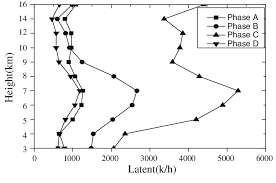## How to Calculate and Solve for Total Latent Heat | Solidification of MetalsThe image above represents total latent heat.

To compute for total latent heat, three essential parameters are needed and these parameters are Density of Solidifying Metal (ρ’), Volume of Casting (V) and Latent Heat of Fusion (Hf).

The formula for calculating the total latent heat:

Q = ρ’VHf

Where:

Q = Total Latent Heat
ρ = Density of Solidifying Metal
V = Volume of Casting
Hf = Latent Heat of Fusion

Let’s solve an example;
Find the total latent heat when the density of solidifying metal is 12, the volume of casting is 14 and the latent heat of fusion is 10.

This implies that;

ρ = Density of Solidifying Metal = 12
V = Volume of Casting = 14
Hf = Latent Heat of Fusion = 10

Q = ρ’VHf
Q = (12)(14)(10)
Q = 1680

Therefore, the total latent heat is 1680 J/Kg.

Calculating the Density of Solidifying Metal when the Total Latent Heat, the Volume of Casting and the Latent Heat of Fusion is Given.

ρ = Q / VHf

Where;

ρ = Density of Solidifying Metal
Q = Total Latent Heat
V = Volume of Casting
Hf = Latent Heat of Fusion

Let’s solve an example;
Find the density of solidifying metal when the total latent heat is 20, the volume of casting is 4 and the latent heat of fusion is 2.

This implies that;

Q = Total Latent Heat = 20
V = Volume of Casting = 4
Hf = Latent Heat of Fusion = 2

ρ = Q / VHf
ρ = 20 / (4)(2)
ρ = 20 / 8
ρ = 2.5

Therefore, the density of solidifying metal is 2.5